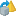You are viewing the RapidMiner Studio documentation for version 9.2 - Check here for latest version

#Principal Component Analysis (RapidMiner Studio Core)

## Synopsis

This operator performs a Principal Component Analysis (PCA) using the covariance matrix. The user can specify the amount of variance to cover in the original data while retaining the best number of principal components. The user can also specify manually the number of principal components.

## Description

Principal component analysis (PCA) is an attribute reduction procedure. It is useful when you have obtained data on a number of attributes (possibly a large number of attributes), and believe that there is some redundancy in those attributes. In this case, redundancy means that some of the attributes are correlated with one another, possibly because they are measuring the same construct. Because of this redundancy, you believe that it should be possible to reduce the observed attributes into a smaller number of principal components (artificial attributes) that will account for most of the variance in the observed attributes.

Principal Component Analysis is a mathematical procedure that uses an orthogonal transformation to convert a set of observations of possibly correlated attributes into a set of values of uncorrelated attributes called principal components. The number of principal components is less than or equal to the number of original attributes. This transformation is defined in such a way that the first principal component's variance is as high as possible (accounts for as much of the variability in the data as possible), and each succeeding component in turn has the highest variance possible under the constraint that it should be orthogonal to (uncorrelated with) the preceding components.

Please note that PCA is sensitive to the relative scaling of the original attributes. This means that whenever different attributes have different units (like temperature and mass); PCA is a somewhat arbitrary method of analysis. Different results would be obtained if one used Fahrenheit rather than Celsius for example.

## Input

•example set (IOObject)

This input port expects an ExampleSet. It is output of the Retrieve operator in the attached Example Process. The output of other operators can also be used as input. It is essential that meta data should be attached with the data for the input because attributes are specified in their meta data. The Retrieve operator provides meta data along with the data. Please note that this operator cannot handle nominal attributes; it works on numerical attributes.

## Output

•example set (IOObject)

The Principal Component Analysis is performed on the input ExampleSet and the resultant ExampleSet is delivered through this port.

•original (IOObject)

The ExampleSet that was given as input is passed without changing to the output through this port. This is usually used to reuse the same ExampleSet in further operators or to view the ExampleSet in the Results Workspace.

•preprocessing model (Preprocessing Model)

This port delivers the preprocessing model, which has information regarding the parameters of this operator in the current process.

## Parameters

• dimensionality_reductionThis parameter indicates which type of dimensionality reduction (reduction in number of attributes) should be applied.
• none: if this option is selected, no component is removed from the ExampleSet.
• keep_variance: if this option is selected, all the components with a cumulative variance greater than the given threshold are removed from the ExampleSet. The threshold is specified by the variance threshold parameter.
• fixed_number: if this option is selected, only a fixed number of components are kept. The number of components to keep is specified by the number of components parameter.
Range: selection
• variance_thresholdThis parameter is available only when the dimensionality reduction parameter is set to 'keep variance'. All the components with a cumulative variance greater than the variance threshold are removed from the ExampleSet. Range: real
• number_of_componentsThis parameter is only available when the dimensionality reduction parameter is set to 'fixed number'. The number of components to keep is specified by the number of components parameter. Range: integer

## Tutorial Processes

### Dimensionality reduction of the Polynomial data set using the Principal Component Analysis operator

The 'Polynomial' data set is loaded using the Retrieve operator. The Covariance Matrix operator is applied on it. A breakpoint is inserted here so that you can have a look at the ExampleSet and its covariance matrix. For this purpose the Covariance Matrix operator is applied otherwise it is not required here. The Principal Component Analysis operator is applied on the 'Polynomial' data set. The dimensionality reduction parameter is set to 'fixed number' and the number of components parameter is set to 4. Thus the resultant ExampleSet will be composed of 4 principal components. As mentioned in the description, the principal components are uncorrelated with each other thus their covariance should be zero. The Covariance Matrix operator is applied on the output of the Principal Component Analysis operator. You can see the covariance matrix of the resultant ExampleSet in the Results Workspace. As you can see that the covariance of the components is zero.